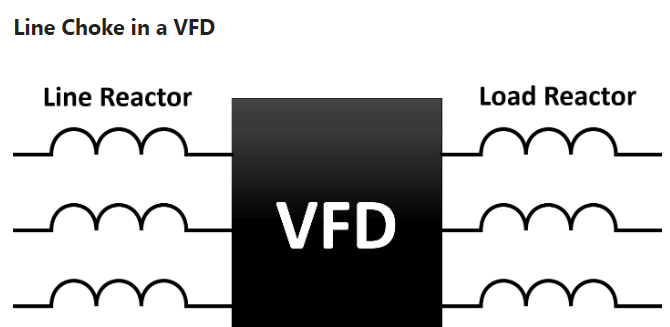## What is a Line Choke or Reactor in a VFD?

Line choke is an inductance connected to the VFD input or output circuit. It is a component that forms a magnetic field as current flows through it and when the current increases, it limits the increment in current by producing a voltage or emf across it that opposes it.

This protects the VFD from unwanted spikes, transients, and harmonics. The reactor is used on either the input side or output side of the VFD.

When a reactor is used on the input side, it is called a line reactor and when it is used on the output side, it is called a load reactor.Refer to the above image. The inductance is shown on the input side and the output side is nothing but the line choke. It is normally used for VFD’s which have a rating of more than 1 KVA. Let us understand its basic working.

It is not always necessary that a current wave be sinusoidal. When it is not sinusoidal, it contains harmonics. Harmonics are a large current distortion.

If you see the basic equation of an inductor, it is – V = L (di/dt)

Here, V is the voltage, L is the inductance of the choke or reactor, and (di/dt) is the rate of change of current.

As the current change rate increases, the voltage too increases in proportional to the inductance.

But, this induced voltage has the opposite polarity to that of the applied voltage. This automatically reduces the rate of current change and limits the current applied.

## Line Choke

One more theory can be understood here for the output side of VFD. As a high switching frequency is produced at the output of VFD, sharp changes in voltage outputs are reflected sometimes.

This high spikes in voltage can damage the motor circuit or even heat the VFD itself. This can be reduced by using a line choke.

The line choke reduces the peak of the voltage waveform and increases the changing rise time of the signal; to stabilize the final output waveform.

The stabilized waveform will indirectly provide limited current and voltage output to the motor.